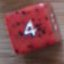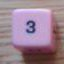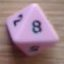Comparing two dice pools Exploding dice

## Comparing two dice pools Exploding dice

Return to topic list

 Wed Jul 20 00:34:32 2016   by   cptnfiskedritt How would you do this correctly?SDICE:=1d8; \"Skill, rank d4 (high) to d20 (low)"AONE:=14; \"Att 1: rank d4 (low) - d20 (high) in increments of 2 (dice size)"ATWO:=14; \"Att 2: rank d4 (low) - d20 (high) in increments of 2 (dice size)"ATHREE:=14; \"Att3: rank d4 (low) - d20 (high) in increments of 2 (dice size)"SKILL:= 1#(accumulate x:=SDICE while x=1) ;count SKILL <= (1d AONE@1d ATWO@1d ATHREE)The idea is to compare one dice (skill) to three other dice acting as a threshold you roll under (attributes). The skill dice can explode. Thu Jul 21 15:52:10 2016   by   wyrdR \ I think this might be what you're after(?)R := 8 ; \"Skill, rank d4 (high) to d20 (low)"A:=  4  ; \"Att 1: rank d4 (low) - d20 (high) in increments of 2 (dice size)"B:= 12  ; \"Att 2: rank d4 (low) - d20 (high) in increments of 2 (dice size)"C:= 20  ; \"Att3: rank d4 (low) - d20 (high) in increments of 2 (dice size)"S:= accumulate x:=(d R) while x=1 ;count S <= ((d A) @ (d B) @ (d C)) Thu Jul 21 15:55:45 2016   by   wyrdR Correction :S:=sum  (accumulate x:=(d R) while x=1) ; \ Assuming you want a total Thu Jul 21 22:10:59 2016   by   cptnfiskedritt Almost. Your first one is what I want. That is to say I want Skill dice compared to the attributes. If the Skill die explodes (rolls up a 1) then a second (third, fourth; however many explosions) Skill die will be rolled and compared to the attributes. Successes are counted (roll Skill dice under attribute dice). Without exploding Skill dice there would be between 0-3 results possible. With exploding skill dice this increases to an infinite amount (until statistically insignificant).The problem I have is the one I get with your program as well. "Non-singleton used with <="I think I understand why I get the error (it compares two sets of variable probability distributions against each other). The error only crops up when I use the "accumulate" function without "sum" or "choose x". Fri Jul 22 10:41:24 2016   by   wyrdR You can only have a "singleton" to the left of <= so without the sum it will error out whenever S explodes.  A singleton is a set of exactly one die, not an empty set and certainly not multiple dice. So the error is absolutely correct!Maybe write in plain English what you're trying to achieve and we can try to work it out.For instance,count S <= ((d A) @ (d B) @ (d C))Means:⒈ Roll an A sided die, a B sided die and a C sided die, and that's our right hand set of <= (RHS)⒉ S is an exploded dice roll which contains one or more dice in the left hand side of <= (LHS)⒊ <= means take away any roll in RHS that is less than the LHS (S), but this breaks whenever S is not a single dice! ⒋ Then we try to count the number of dice left in the RHS, keeping only those higher or equal to S. But if S is more than one value, which is it?! The result could only be 0 to 3 or an error. Fri Jul 22 10:55:04 2016   by   wyrdR \ Third attempt...R := 8 ; \"Skill, rank d4 (high) to d20 (low)"A:=  4  ; \"Att 1: rank d4 (low) - d20 (high) in increments of 2 (dice size)"B:= 12  ; \"Att 2: rank d4 (low) - d20 (high) in increments of 2 (dice size)"C:= 20  ; \"Att3: rank d4 (low) - d20 (high) in increments of 2 (dice size)"Att  :=  (d A) @ (d B) @ (d C)  ;S:= accumulate x:=(d R) while x=1 ;count (  foreach  t in S  do (  t  <= ( Att  )  )  ) Fri Jul 22 22:12:13 2016   by   Cptnfiskedritt This looks exactly like it! And it was that simple. Thanks a lot though! This was exactly what I was looking for, and sorry for not putting it more plainly. Case solved!

Return to topic list

New message:
 Topic: Posted by:

Type the values of the dice shown below:Return to topic list